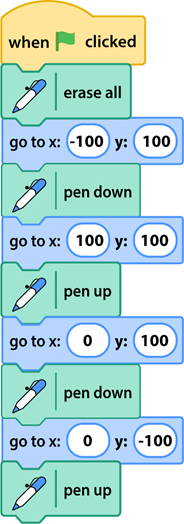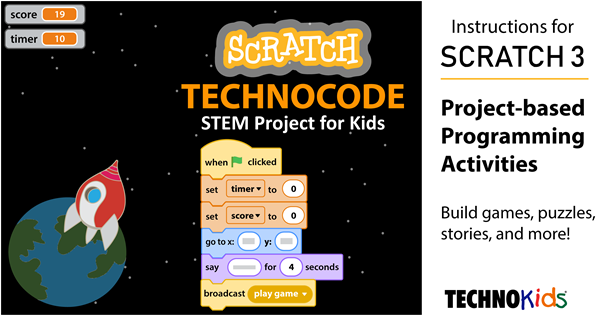# Scratch Activities, About the Stage, Draw with the Pen

When completing Scratch activities your students will benefit from understanding ordered pairs. This is a mathematical concept that is often not taught until the higher grades. However, it is knowledge that will help young programmers. It can be used to place sprites on the stage by setting the x and y values. It also helps when debugging scripts to determine why the positioning or movement is incorrect.

NOTE: The information in this blog article is from TechnoCode. TechnoCode includes Scratch activities that are ideal for beginners.

## X and Y Coordinates

When you place a character on the stage, the Sprite pane shows x and y coordinates. They tell where the sprite is on the stage. If you drag the sprite, the numbers change because the position is now different.

Many Scratch coding blocks list x and y values. These coding blocks can be used to tell a character where to go on the stage.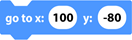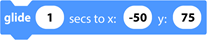How do you know the value of x and y? Where do you get the numbers?

You cannot see it – but the Scratch stage is divided into four parts. This is done using two number lines.

• The x number line goes from left to right. It tells where a sprite is on the stage horizontally.
• The y number line goes from top to bottom. It tells where a sprite is on the stage vertically.
• The center of the stage is where the two number lines meet.

## What Is the X and Y Value?

Each part of the stage is made up of dots. Each dot has an x value and a y value that tell its location on the stage.

• The x value tells you how many steps to move right or left from the center point. If the number is positive, move right. If it is negative, move left
• The y value tells you how many steps to move up or down from the center point. If the number is positive, move up. If it is negative, move down.

Look at the picture below. Where is the dot on the stage? First, count across to get the x value. Then count up or down to get the y value. It is at x: 150, y: 100.

## Where Is the Sprite on the Stage?

The x number line starts at -240 and ends at 240.

The y number lines starts at -180 and ends at 180.

By setting the x and y value for a coding block, you can place a sprite at a specific spot on the stage. For example:

center of stage    x: 0 and y: 0

right edge of stage    x: 240 and y: 0

left edge of stage    x: -240 and y: 0

top edge of stage    x: 0 and y: 180

bottom edge of stage    x: 0 and y: -180

## Scratch Activities for Kids – Use the Pen to Learn About X and Y

This activity is an excerpt from TechnoCode. The Scratch lesson includes four drawing activities designed to help students comprehend X and Y coordinates.

Drawing in Scratch is like doing a dot-to-dot puzzle. To draw a picture, build a script that puts the pen down at the first dot. Then move the pen from one dot to next. Try it!

Look at the picture of the Scratch stage. What are the x and y coordinates for each dot?

### How to Draw a Square in Scratch

1. Start a new Scratch project. Name it square.
2. Select a sprite to use as a drawing tool.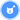3. Resize the sprite to make it small.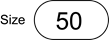4. Delete Sprite 1.
5. Click Add Extension.Select Pen.
6. Build the script:

About the script: Start when Go is clicked. Move to the first dot. Put the pen down to draw. Draw from one dot to another. See the square for 3 seconds. Erase the square.

## Scratch Activities – Sketch a Drawing

Drawing a picture in Scratch is like doing a dot-to-dot puzzle. In a dot-to-dot, each dot is numbered. A line joins one dot to the next. The lines form a picture.

Use your skills to make your own picture. Pick a suggestion or come up with your own idea.

• triangle
• rectangle
• house
• letter (T or E)
• zig zag line

Download the Scratch Stage Worksheet. Sketch your idea for a drawing. Circle the “dot” at the beginning and at the end of each line. List the x and y values for each “dot” in the order they should be drawn.

TIP: Write pen up after a “dot” or x y value that should not have a line drawn to the next “dot” or x y value in the list.